# Applications of distribution law

## Applications of distribution law

– There are numerous applications of distribution law in the laboratory as well as in industry.

– Here we will discuss some more important ones by way of recapitulation.

## (1) Solvent Extraction

– This is the process used for the separation of organic substances from aqueous solutions.

– The aqueous solution is shaken with an immiscible organic solvent such as ether (or benzene) in a separatory funnel.

– The distribution ratio being in favour of ether, most of the organic substance passes into the ethereal layer.

– The ethereal layer is separated and ether distilled off. Organic substance is left behind.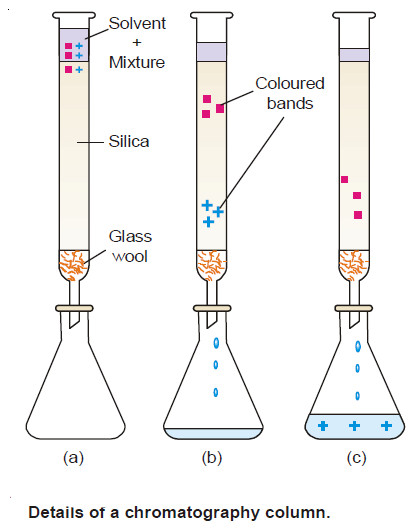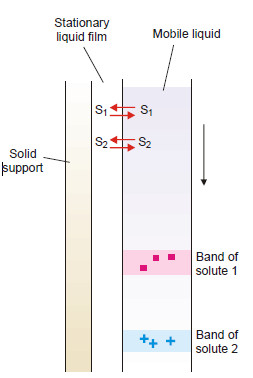In partition chromatography, the liquid film on the solid support is the stationary phase. The second phase is an immiscible solvent flowing down. Each solute (S1 and S 2) is distributed between the stationary liquid film and the mobile liquid phase. The solute (say S2 ) with higher distribution ratio comes down first.

– The process of extraction is more efficient if the solvent is used in a number of small portions
than in one whole lot.

– Thus multiple extraction is preferred to simple extraction.

– The multiple extraction is also the basis of the industrial counterflow solvent extraction. A dilute solution of the substance enters at the top of a column or tower.

– At the same time the extracting solvent enters at the bottom.

– As the aqueous solution and the solvent pass each other, the solute distributes itself in favour of the solvent.

– Since the process of extraction is repeated many times at different levels in the tower, maximum quantity of substance is removed in the solvent.

## (2) Partition Chromatography

– This is a modern technique of separating a mixture of small amounts of organic materials.

– A paste of the mixture is applied at the top of a column of silica soaked in water. Another immiscible solvent, say hexane, is allowed to flow down the column.

– Each component of the mixture is partitioned between the stationary liquid phase (water) and the mobile liquid phase (hexane).

– The various components of the mixture are extracted by hexane in order of their distribution coefficients.

– Thus the component with the highest distribution coefficient is first to move down in the flowing hexane which is collected separately.

– Similarly, a component with a lower distribution ratio comes down later and is received in another vessel.

## (3) Desilverization of Lead (Parke’s Process)

– When molten zinc is added to molten lead containing silver (argentiferous lead), zinc and lead form immiscible layers and silver is distributed between them. Since the distribution ratio is about 300 in favour of zinc at 800º C, most of silver passes into the zinc layer.

– On cooling the zinc layer, an alloy of silver and zinc separates. The Ag-Zn alloy is distilled in a retort when zinc passes over leaving silver behind.

– The lead layer still contains unextracted silver. This is treated with fresh quantities of molten zinc to recover most of the silver.

## (4) Confirmatory Test for Bromide and Iodide

– The salt solution is treated with chlorine water. Small quantity of bromine or iodine is thus liberated.

– The solution is then shaken with trichloromethane (chloroform). On standing chloroform forms the lower layer.

– The free bromine or iodine being more soluble in chloroform concentrates into the lower layer, making it red for bromine and violet for iodine.

## (5) Determination of Association

– When a substance is associated (or polymerized) in solvent A and exists as simple molecules in solvent B, the Distribution law is modified as: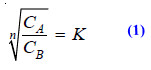when n is the number of molecules which combine to form an associated molecule.

– Suppose benzoic acid is associated to bimolecules, (C6H5COOH)2, in benzene and is present as simple molecules in aqueous layer. Then equation (1) can be written as follows where n = 2.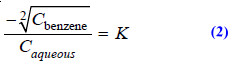– The concentrations in benzene and aqueous layer are determined experimentally. If the under root of the concentration in benzene divided by the concentration in aqueous layer gives a constant value benzoic acid exists as bimolecules (dimer) in benzene.

## (6) Determination of Dissociation

– Suppose a substance X is dissociated in aqueous layer and exists as single molecules in ether.

– If x is the degree of dissociation (or ionisation), the distribution law is modified as: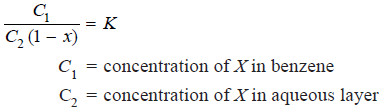– The value of x can be determined from conductivity measurements, while C1 and C2 are found experimentally.

– Thus the value of K can be calculated. Using this value of K, the value of x for any other concentrations of X can be determined.

## (7) Determination of Solubility

Suppose the solubility of iodine in benzene is to be determined. Iodine is shaken with water and benzene. At equilibrium concentrations of iodine in benzene (Cb) and water (Cw) are found experimentally and the value of distribution coefficient calculated.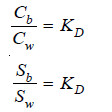where Sb = solubility in benzene;

and Sw = solubility in water.

– If the solubility of iodine in water (Sw) is known, the solubility in benzene can be calculated.

## (8) Deducing the Formula of a Complex Ion  (I3– )

Some iodine is added to a solution of KI and the reaction mixture shaken with benzene.

(a) The [I2] in water layer can be found knowing the value of KD (determined separately) and concentration of iodine in benzene (determined by titration against thiosulphate).

(b) The total concentration of iodine, [I2] + [I3] in water layer is found by titration against thiosulphate. Knowing [I2] from (a),  [I3− ] can be calculated.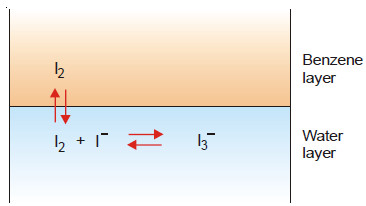(c) The initial concentration of KI taken is represented by the equilibrium concentrations [I] + [I3 ]. Knowing  [I3−] from (b), [I] can be found.

– Substituting the above values of concentrations in the law of Mass Action equation of the reaction in water layer,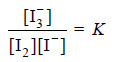– the value of equilibrium constant K can be calculated.

– If it comes out to be constant for different concentrations of iodine, it stands confirmed that the formula of the complex I3 , which we assumed is correct.

## (9) Distribution Indicators

– In iodine titrations, the end point is indicated by adding starch suspension which turns blue.

– A greater sensitivity can be obtained by using what we may call (Distribution Indicator).

– A few drops of an immiscible organic solvent such as chloroform (or carbons tetrachloride) is added to the solution.

– The bulk of any iodine present passes into the organic layer and imparts intense violet colour to it.

– so Applications of distribution law are discussed.